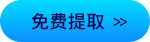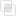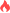python中K-NN算法是什么？2021-03-01 15:10:32346浏览 · 0收藏 · 0评论K Nearest Neighbor算法⼜叫KNN算法， 这个算法是机器学习⾥⾯⼀个⽐较经典的算法， 总体来说KNN算法是相对⽐

1、定义

2、基本流程

（1）计算已知类别数据集中的点与当前点之间的距离2)按距离递增次序排序

（2）选取与当前点距离最小的k个点

（3）统计前k个点所在的类别出现的频率

（4）返回前k个点出现频率最高的类别作为当前点的预测分类

3、实例

```from sklearn.datasets import load_iris
from sklearn.model_selection import train_test_split
from sklearn.preprocessing import StandardScaler
from sklearn.neighbors import KNeighborsClassifier

# 1.获取数据
iris = load_iris()

# 2.数据基本处理：训练集的特征值x_train 测试集的特征值x_test 训练集的⽬标值y_train 测试集的⽬标值y_test，
'''
x: 数据集的特征值
y: 数据集的标签值
test_size: 测试集的⼤⼩， ⼀般为float
random_state: 随机数种⼦,不同的种⼦会造成不同的随机采样结果。 相同的种⼦采样结果相同
'''
x_train, x_test, y_train, y_test = train_test_split(iris.data, iris.target, test_size=0.2, random_state=22)

# 3.特征工程 - 特征预处理
transfer = StandardScaler()
x_train = transfer.fit_transform(x_train)
x_test = transfer.transform(x_test)

# 4.机器学习-KNN
# 4.1 实例化一个估计器
estimator = KNeighborsClassifier(n_neighbors=5)
# 4.2 模型训练
estimator.fit(x_train, y_train)

# 5.模型评估
# 5.1 预测值结果输出
y_pre = estimator.predict(x_test)
print("预测值是:\n", y_pre)
print("预测值和真实值的对比是:\n", y_pre==y_test)

# 5.2 准确率计算
score = estimator.score(x_test, y_test)
print("准确率为:\n", score)```python中pyg2plot如何使用？python pyg2plot的原理python在协程中增加任务python常见BUG的介绍和整理python如何在CSV中添加标题行python如何对多个CSV文件进行读取Python装饰器的应用场景344

Python map接收参数的探究369

Python如何自定义类继承threading.Thread168